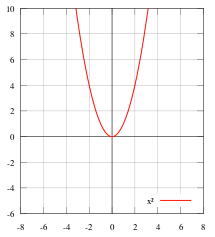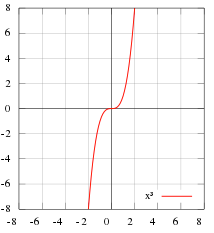# Even and Odd Functions: Definition, Test, Integrating

Contents:

## What are Even and Odd Functions?

Even and odd functions have special symmetries about the origin or y-axis.

• A function is even if it is symmetric about the vertical y-axis; if this is the case, f(-x) = f(x) for every x in the domain.
• A function is odd if it is symmetric about the origin. In this case, f(-x) = -f(x) for every x in the domain.

## Examples of Even and Odd Functions

The parabola f(x) = x2 is an example of an even function. As you can see in the image below, it is symmetric about the vertical y axis.The function f(x) = x3, on the other hand, is an example of an odd function. It is symmetric about the origin.Since the definition of even and odd are not mutually exclusive, it is possible to have a function that is both even and odd. For such a function, both f(-x) = f(x) and f(-x)= -f(x) are true. Substituting those equations into each other, we find that, for these functions, f(x) = -f(x). There is only one way that can be true: if f(x) = 0. So the function f(x) = 0 is the one and only function that is both even and odd.

## Properties of Odd and Even Functions

Here are some basic properties of odd and even functions that are worth remembering:

• The sum of two odd functions is odd, and the sum of two even functions is even (note that this is not the same as the rule for even and odd integers)
• The sum of an even and an odd function is neither even nor odd unless one or both of them is actually equal to zero.
• The difference of two even functions is even; likewise, the difference of two odd functions is odd.
• The product of two even functions is another even function, and the product of two odd functions is an even function also.
• The product of an even function and an odd function is an odd function.

## Test for Even and Odd Functions

This simple test states:

A function y – f(x) is even if f(-x) = f(x)
A function y – f(x) is odd if f(-x) = f(x)

Example question #1: Is the function f(x) = x3 – x odd or even?

Solution:
Step 1: Replace any “x” in the function with “-x”:
f(-x) = (-x)3 – (-x)

Step 2: Solve with algebra.The goal is to get the function in a form that is either the original function, x3 – x, or its negative -(x3 – x),

• f(-x) = (-x)3 – (-x) =
• = -x3 + x

The result, -x3 + x, is the negative of the function so this function is odd.

Example question #2: Is the function f(x) = 2 + cos(x) odd or even?

Solution:
Step 1: Replace any “x” in the function with “-x”:
f(-x) = 2 + cos(-x)

Step 2: Solve with algebra.The goal is to get the function in a form that is either the original function, x3 – x, or its negative -(x3 – x),

• f(-x) = 2 + cos(x)*

The result, 2 + cos(x) is the original function, so this is an even function.
One of the Trigonometric identities for negatives states that -cos(x) = cos(x).

## Integration of Even and Odd Functions

The theorem of “Integration of Even and Odd Functions” is a way to find integrals for odd and even functions. It’s a method that makes some challenging definite integrals easier to find. In order to use it, you have to be evaluating a function over an interval that’s either symmetric about the origin or symmetric about the y-axis .

In order to use this theorem to simplify finding a definite integral:

1. Your closed interval must be in the form [-a, a]. In other words, your interval must have the same number “a” like [-3, 3] or [-π, π].
2. You must have an even or odd function. If you aren’t sure use the Test for Even and Odd Functions to check before continuing.

## Integration of Even and Odd Functions: Even Example

1. Even functions Rule:Example question: Find the follow integral:We can multiply the highlighted area by 2 to get the area of the entire region because the function is symmetrical about the y-axis.
Step 1: Rewrite the integral to cover the positive half of the region (shown in the above image). For this example, instead of integrating from -1 to 1, we’re integrating from 0 to 1:Step 2: Solve the integral (I used Symbolab’s calculator):
Solving the integral, we get 8/7 ≈ 1.14285.

Step 3: Multiply the solution from Step 2 by 2:
8/7 * 2 = 16/7 ≈ 2.28571.

That’s it!

## 2. Odd functions Definite Integral Example

This is the easiest definite integral you’ll ever calculate (actually, you don’t even have to do any calculations…read on!).
The formula is:This part of the theorem is easier than for even functions. It simply states that if your function is symmetric about the origin for interval [-a, a], the definite integral is going to be zero.Graph of an odd function. The two blue regions are identical in area but opposite in sign so cancel each other out,

## References

Smith, Ken W. Elementary Functions Part 1: Lecture 1.4a, Symmetries of Functions. Retrieved from https://www.shsu.edu/~kws006/Precalculus/1.4_Function_Symmetries_files/1.4%20FunctionSymmetries%20slides%204to1.pdf on August 10, 2019.
Siktar, Joshua. Algebraic Analysis of Functions: Even and Odd Functions. Retrieved from https://opencurriculum.org/5507/even-and-odd-functions/ on August 10, 2019.

CITE THIS AS:
Stephanie Glen. "Even and Odd Functions: Definition, Test, Integrating" From StatisticsHowTo.com: Elementary Statistics for the rest of us! https://www.statisticshowto.com/even-and-odd-functions/

Subscribe to our Statistics How To channel on YouTube!